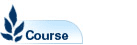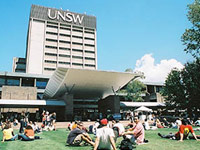# Engineering Mathematics 2E - MATH2019

Faculty: Faculty of Science

Course Outline: http://www.maths.unsw.edu.au/

Campus: Sydney

Units of Credit: 6

Indicative Contact Hours per Week: 6

Enrolment Requirements:

Prerequisite: MATH1231 or MATH1241 or MATH1251.

Excluded: MATH2018, MATH2020, MATH2029, MATH2059, MATH2120, MATH2121, MATH2130, MATH2221

Tuition Fee: See Tuition Fee Schedule

Further Information: See Class Timetable

View course information for previous years.

## Description

Partial differentiation and applications, vector algebra, double integrals, ordinary differential equations, introduction to vector field theory, extrema of functions of 2 variables, matrices and their applications, Laplace transforms, Fourier series, partial differential equations and their solution for selected physical problems.

Note: Available only to students for whom it is specifically required as part of their program. MATH2018 is equivalent to MATH2019.